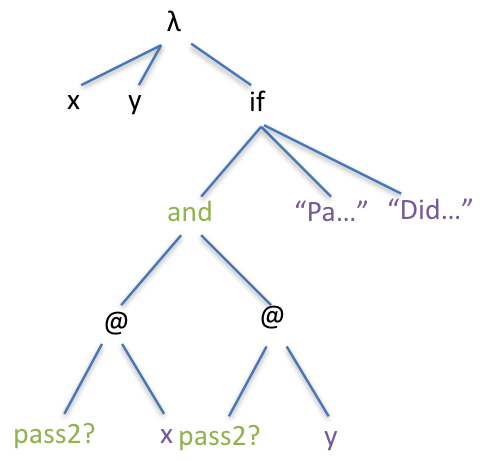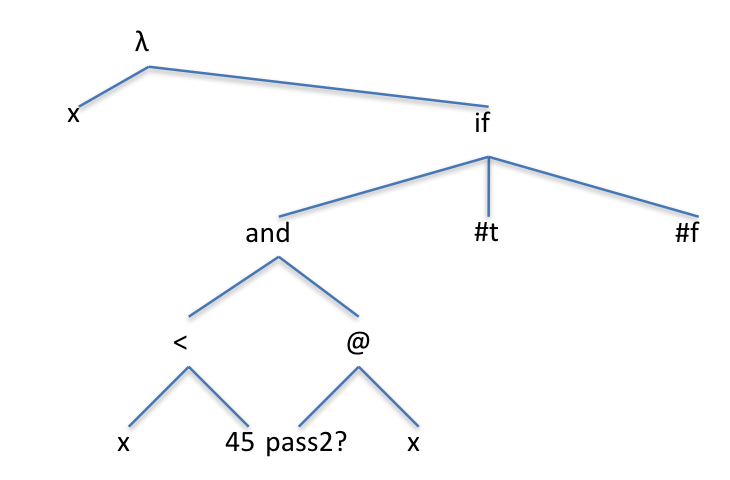# Using Conditionals In Functions

We can use IF to make decisions in our programs. Here we display either “pass” or “fail” depending on the grade that is passed to this function;

```(define pass?
(lambda (x)
(if (> = x 40) "pass" "fail")
)
)
```

Notice the indentation of the brackets. This doesn’t change the functionality, but it makes it easier to read — another example of syntax versus semantics. pass? takes one parameters and, if it is greater than or equal to 40, returns the word “pass”.

Here’s a similar function. This one only returns #t or #f:

```(define pass2?
(lambda (x)
(if (> = x 40) #t #f)
)
)

> (pass? 30)
"fail"
> (pass2? 30)
#f```

Although it may seem like pass? is more useful as it returns something readable by us humans, it turns out that it is actually less useful because of this. pass2? is what is known as tightly coupled, that is, all the instructions in it are related to one single task, in this case, testing the value passed in and returning #t or #f. It is also reusable, which makes it a lot more useful than pass2?. In fact, most of the functions we’ve looked at throughout this course, such as sqrsqrt, etc. are tightly coupled and reusable. Remember how back in the section on lifetime diagrams we said that defined variables and functions became part of the language? The reason that some of these are so useful is because they are reusable.

There’s also a second problem with pass?. Consider

```(if
(and (pass? 25) (pass? 60))
"passed both"
"didn't pass both")
)
)
```

When we evaluate the calls to pass? the and part becomes:

(and “fail” “pass”)

This is where we have a problem, because, as we saw in the previous section, all strings are considered true, and remember, and returns the last true value it is given, otherwise it returns false. This means that it returns “pass”, which is wrong. Look at this example; here everything works as we would expect, because pass2? returns a Boolean value.

```(define passBoth
(lambda (x y)
(if
(and (pass2? x) (pass2? y))
"passed both"
"didn't pass both")
)
)
```

Here’s the AST for this function. Notice how we have two @ nodes inside the predicate where we’re testing the conditions; this is because this is a function we wrote ourselves rather than a built in function.Using pass2? inside another function

Let’s look at another example:

```(define scrape
(lambda (x)
(if
(and (< x 45) (pass2? x))
#t
#f)
)
)
```

Here we reuse pass2? again, combining with another test inside the body of the function to see if the grade was just barely a pass. Notice the difference in the AST — we still have @ for pass2?, but not for the (< x 45) test.Mixing built-in and user defined tests in the predicate

Here’s one more version of a function that tests if a grade is a pass. This one also returns a Boolean value but doesn’t even have an IF

```(define pass3?
(lambda (x)
(> = x 40)
)
)

```

How does this one work? It evaluates (>= x 40) which gives a Boolean, and simply returns that, so it’s much more efficient.

Let’s look at one final example. We want to write a function called high-odd, which returns true if a number is greater than 100 and is also odd.

We could all this in one function, but let’s spread it out across two to make it easier to read. We’ll first check if the number is odd, and to do this we’ll use a built in Racket function called integer? which returns #t if its argument is an integer (a whole number with no decimal point) and returns #f otherwise. Here’s our odd function:

```(define odd
(lambda (x)
(not (integer? (/ x 2)))
)
)
```

Satisfy yourself that you understand how it works. It divides the parameter (x) by 2 and, if x is odd then integer? would return false (because odd numbers divided by two will have a decimal point), so we return the opposite of that using not because our test should return true if x is an odd number.

```(define high-odd
(lambda (x)
(and (> x 100) (odd x))
)
)

(define high-odd2
(lambda (x)
(if (> x 100) (odd x) #f)
)
)
```

high-odd needs to do two tests; the first checks if x is above 100 and the second that it is an odd number. In fact, we’ve given two versions. The only difference is that high-odd2 will only call odd if it actually needs to. Intuitively, this seems like a more efficient piece of code, after all, it seems like there will be less code executed, right? Actually, it turns out that high-odd is more efficient, why? Remember how and works — if it encounters a false value, it stops evaluating and returns that value, so if x isn’t greater than 100, it doesn’t check if is odd or not, because it doesn’t matter.

However, if x is greater than 100, high-odd2 makes another function call. This is a problem because it incurs function overhead, that is, it takes time to set up its local variable, so it is slightly slower. This make very little difference when we’re only calling the function once, but if we were to call it a billion times, this would all add up.

In the next section, when we create functions that will be called hundreds of times, we’ll see up close and personal just how much of a difference these extra function calls can have.